# 初识Python之面向对象篇（下）

+关注继续查看
'''生成器'''
def fib(max):
a,b=1,1
while a <max:
yield a
a,b=b,a+b
for n in fib(15):
print(n)

def xie():
print("等待接收任务......")
while True:
data=(yield )
print("收到任务：",data)
def producer():
c=xie()
c.__next__()
for i in range(5):
print("发送一个任务......","任务%d"%i)
c.send("任务%d"%i)
if __name__=="__main__":
producer()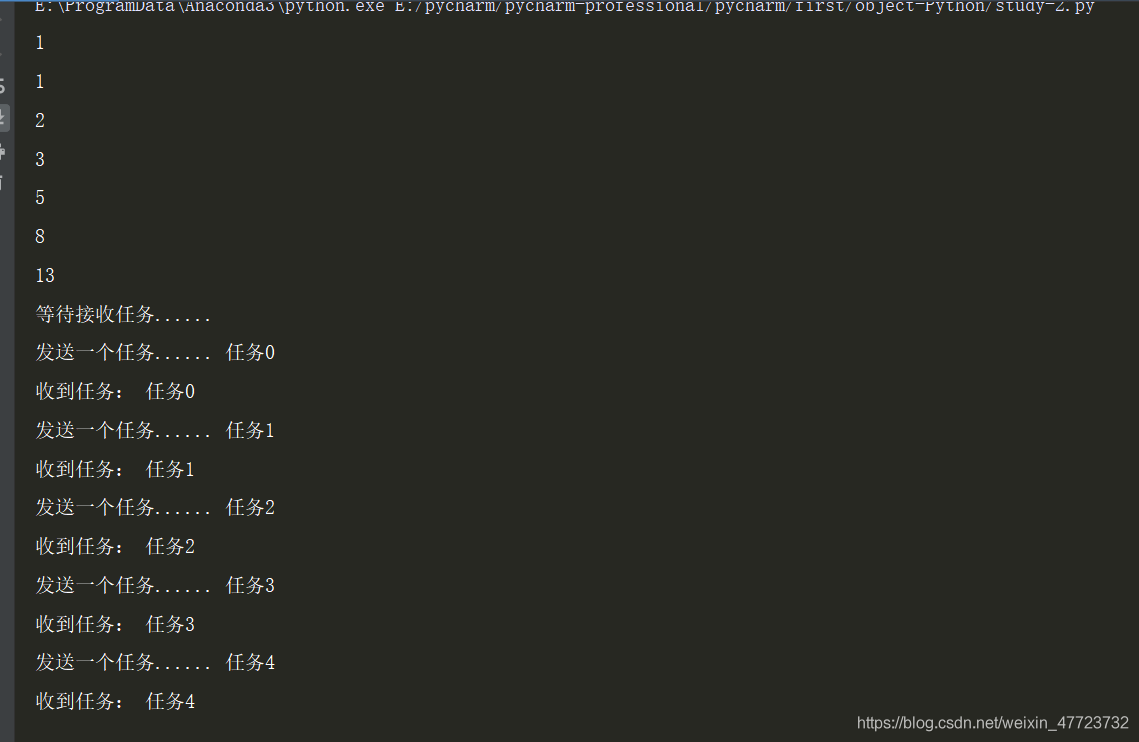'''装饰器'''
'''装饰器可以解决我们在实际开发当中，减轻我们代码的重复率，提高效率'''
def window(run):
def wrapper(*args,**bian):#定义了一个包装器函数
print("植入木马中......")
run(*args,**bian)
print("木马已经渗入......")
return wrapper
@window
def worm(x):
a=[]
for i in range(x):
a.append(i)
print(a)
@window
def str(name):
print("hello",name)
if __name__=="__main__":
worm(10)
print()
str("h7n9病毒")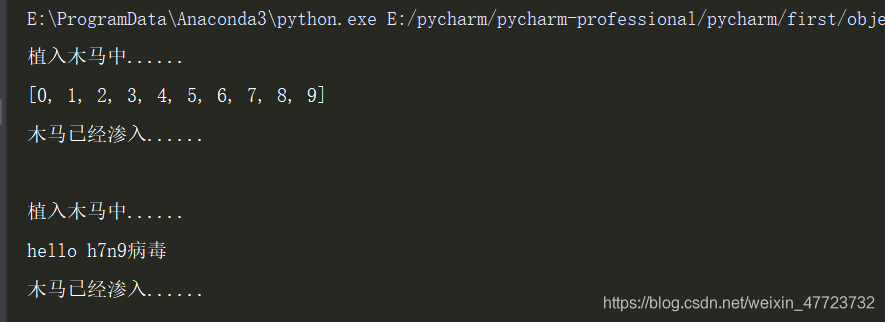def deco(func):
def _deco(a,b):
print("在函数myfunc（）之前被调用。")
ret=func(a,b)#这里必须要将装饰器里面的参数实例化，不然不会产生效益
print("在函数myfunc()之前被调用，结果是：%s"%ret)
return ret
return _deco
@deco
def myfunc(a,b):
print("函数myfunc(%s,%s)被调用！"%(a,b))
return a+b
myfunc(4,2)
myfunc(10,16)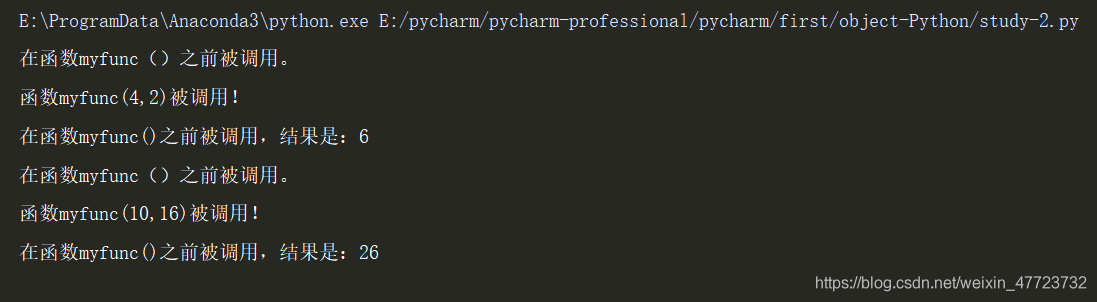# 使用装饰器装饰类
def zz(myclass):
class InnerClass:
def __init__(self,z=0):
self.z=z
self.wrapper=myclass()
def position(self):
self.wrapper.position()
print('z轴坐标：',self.z)
return InnerClass
@zz
class coordination:
def __init__(self,x=0,y=0):
self.x=x
self.y=y
def position(self):
print("x轴坐标：",self.x)
print("y轴坐标：",self.y)
if __name__=="__main__":
coor=coordination()
coor.position()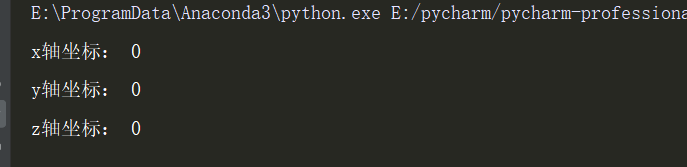'''命名空间'''
'''涉及到全局变量和局部变量，这里必须要明确两个访问函数
local（）可以访问局部命名空间
globals()函数可以访问全局变量
'''
def func(i,str):
x=123321
print(locals())
func(1,"wangxiaowang")#包括了调用函数的复杂语法书写，简化了代码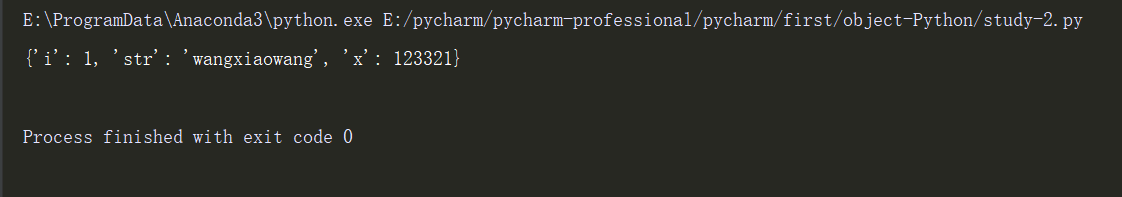'''闭包'''
def func(name):
def inner_func(age):
print("name",name,"\t","age",age)
return inner_func
bb=func("笑傲江湖")#调用func的时候就开始产生了一个闭包inner_func，里面有自己的name，就算函数执行完后，name依然会存在的
bb(19)

def delay_fun(x,y):
def caculator():#使用嵌套函数，实现延迟求和功能
return x+y
return caculator
if __name__=="__main__":
print("返回一个可以求和的函数，但是不去求和！")
msum=delay_fun(3,4)
print()
print("返回一个计算值！")
print(msum())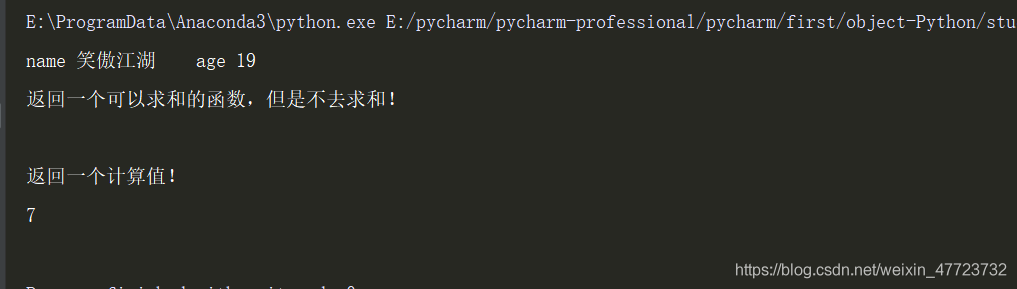'''装饰器与闭包'''
def deco(func):
def _deco():
print("函数闭包实现......")
func()#这里才是调用了，所以此函数中只有该函数是实例化了，里面的闭包其实没有调用和打印
print("函数闭包实现中......")
return _deco#只是返回了它的值，并没有调用
@deco#f=deco(f)
def f():
print("使用装饰器实现中......")
if __name__=="__main__":
f()#知道第二次才返回_deco的值，也就是打印了，这个就是闭包的神奇之处。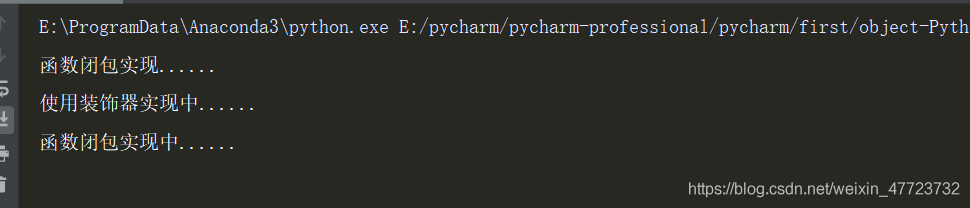'''使用闭包定义泛型函数'''

def fan(a,b):
def afan(x):
return a* x+b
return afan
if __name__=="__main__":
fan23=fan(2,3)
fan25=fan(2,5)
print("调用函数实现：",fan23(4))
print("调用函数熟悉：",fan25(6))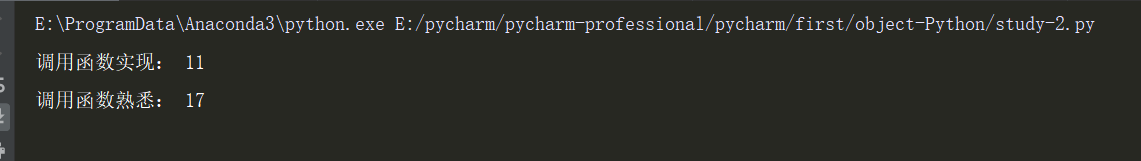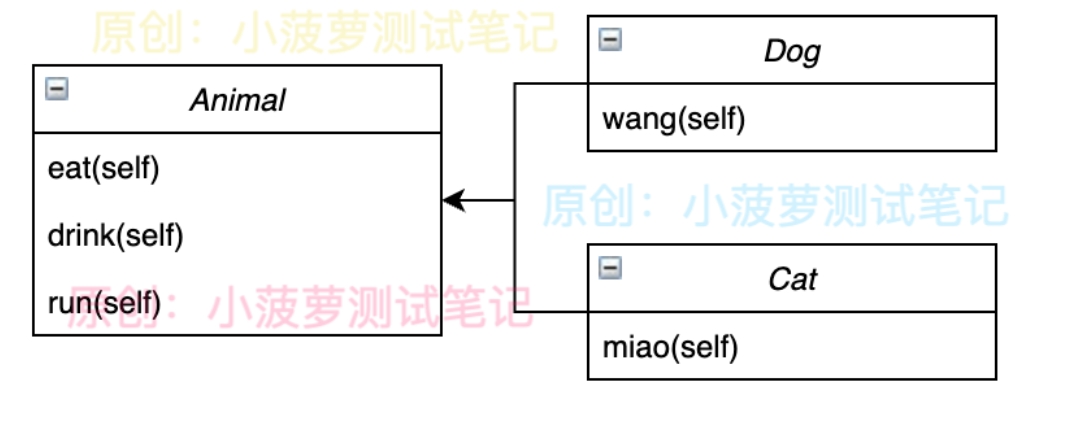Python - 面向对象编程 - 三大特性之继承（下）
Python - 面向对象编程 - 三大特性之继承（下）
12 0python 面向对象
python 面向对象思想
33 0【python】面向对象
1.简单写个例子，因为之前基本没写过python OOP。先写个父类 class Animal: # 构造方法 def __init__(self, name): self.
923 0python-面向对象之继承

935 01023 0Python 面向对象

866 0python面向对象，类
951 0python面向对象之类成员
1006 0python面向对象
701 0723 0

268

0